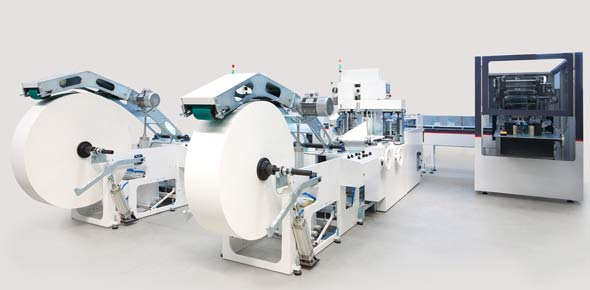# Machine Organization & Assembly Language

7 Questions | Attempts: 425SettingsMachine organization and assembly language, computer science

• 1.
One of the shortcomings of the “signed magnitude” method of representing negativenumbers in binary code is:
• A.

There are two codes for the number zero

• B.

There is no operation for subtraction

• C.

There is no simple test to distinguish positive and negative numbers

• D.

The operation of addition requires complex rules

• E.

Both a and d

• 2.
What is the representation of the (decimal) number –1 in 4 bit 1’s complement format?
• A.

1001

• B.

1011

• C.

1111

• D.

1110

• E.

0001

• 3.
The total number of items representable by a 4 byte binary word (using conventionalabbreviations) is:
• A.

16G

• B.

4G

• C.

32M

• D.

16M

• E.

8k

• 4.
Using two's complement encoding, subtract 11100 from 01101, and report the result as a5-bit two's complement binary number
• A.

101001

• B.

01001

• C.

00100

• D.

10101

• E.

10001

• 5.
Convert the 8-bit two's complement number 10001111 into decimal.
• A.

-241

• B.

-113

• C.

+143

• D.

-143

• E.

+112

• 6.
Assembly Language instruction sets can be categorized into three basic types ofinstruction:
• A.

Operations, Data Movement and Control

• B.

Direct, Register, and Indirect

• C.

High Level, Assembly, and Machine

• D.

Signed magnitude, one's complement, and two's complement

• E.

• 7.
A label in assembly language code is:
• A.

An abbreviation for an instruction

• B.

A symbolic representation of a memory location

• C.

Just a visual reminder for the programmer, ignored by the assembler.

• D.

A temporary name given to a variable

• E.

An adhesive sticker placed on the front page of the code

## Related TopicsBack to top
×

Wait!
Here's an interesting quiz for you.